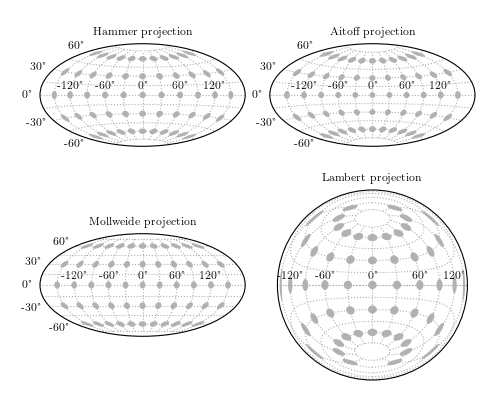# Spherical Projections¶

Figure 1.14.

Four common full-sky projections. The shaded ellipses represent the distortion across the sky: each is projected from a circle of radius 10 degrees on the sphere. The extent to which these are distorted and/or magnified shows the distortion inherent to the mapping.```
```
```# Author: Jake VanderPlas
#   The figure produced by this code is published in the textbook
#   "Statistics, Data Mining, and Machine Learning in Astronomy" (2013)
#   To report a bug or issue, use the following forum:
import numpy as np
from matplotlib import pyplot as plt
from astroML.plotting import plot_tissot_ellipse

#----------------------------------------------------------------------
# This function adjusts matplotlib settings for a uniform feel in the textbook.
# Note that with usetex=True, fonts are rendered with LaTeX.  This may
# result in an error if LaTeX is not installed on your system.  In that case,
# you can set usetex to False.
if "setup_text_plots" not in globals():
from astroML.plotting import setup_text_plots
setup_text_plots(fontsize=8, usetex=True)

#------------------------------------------------------------
# generate a latitude/longitude grid
circ_long = np.linspace(-np.pi, np.pi, 13)[1:-1]
circ_lat = np.linspace(-np.pi / 2, np.pi / 2, 7)[1:-1]
radius = 10 * np.pi / 180.

#------------------------------------------------------------
# Plot the built-in projections
plt.figure(figsize=(5, 4))
left=0.08, right=0.95,
bottom=0.05, top=1.0)

for (i, projection) in enumerate(['Hammer', 'Aitoff', 'Mollweide', 'Lambert']):
ax = plt.subplot(221 + i, projection=projection.lower())

ax.xaxis.set_major_locator(plt.FixedLocator(np.pi / 3
* np.linspace(-2, 2, 5)))
ax.xaxis.set_minor_locator(plt.FixedLocator(np.pi / 6
* np.linspace(-5, 5, 11)))
ax.yaxis.set_major_locator(plt.FixedLocator(np.pi / 6
* np.linspace(-2, 2, 5)))
ax.yaxis.set_minor_locator(plt.FixedLocator(np.pi / 12
* np.linspace(-5, 5, 11)))

ax.grid(True, which='minor')